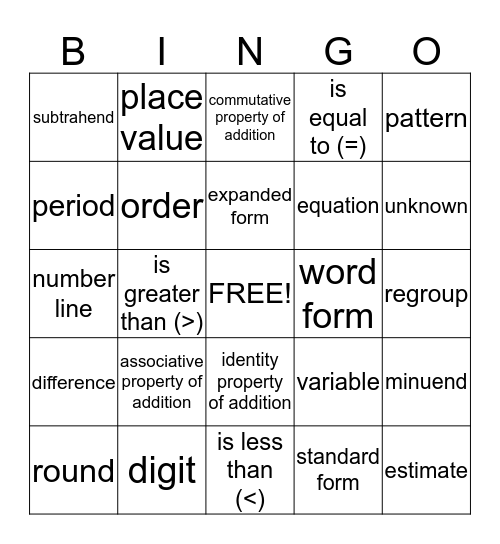Math Chapters 1 and 2 VocabularyThis bingo card has a free space and 24 words: associative property of addition, digit, expanded form, round, pattern, subtrahend, period, place value, word form, identity property of addition, equation, number line, variable, minuend, order, standard form, regroup, commutative property of addition, unknown, is greater than (>), estimate, difference, is equal to (=) and is less than (<).

Play Online